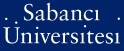# Bilinear optimality constraints for the cone of positive polynomials

Rudolf, Gabor and Noyan, Nilay and Papp, David and Alizadeh, Farid (2011) Bilinear optimality constraints for the cone of positive polynomials. Mathematical Programming, 129 (1). pp. 5-31. ISSN 0025-5610 (Print) ; 1436-4646 (Online)

This is the latest version of this item.

Full text not available from this repository.

Official URL: http://dx.doi.org/10.1007/s10107-011-0458-y

## Abstract

For a proper cone K subset of R(n) and its dual cone K* the complementary slackness condition < x, s > = 0 defines an n-dimensional manifold C(K) in the space R(2n). When K is a symmetric cone, points in C(K) must satisfy at least n linearly independent bilinear identities. This fact proves to be useful when optimizing over such cones, therefore it is natural to look for similar bilinear relations for non-symmetric cones. In this paper we define the bilinearity rank of a cone, which is the number of linearly independent bilinear identities valid for points in C(K). We examine several well-known cones, in particular the cone of positive polynomials P(2n+1) and its dual, and show that there are exactly four linearly independent bilinear identities which hold for all (x, s) is an element of C(P(2n+1)), regardless of the dimension of the cones. For nonnegative polynomials over an interval or half-line there are only two linearly independent bilinear identities. These results are extended to trigonometric and exponential polynomials. We prove similar results for Muntz polynomials.

Item Type: Article Optimality conditions; Positive polynomials; Complementarity slackness; Bilinearity rank; Bilinear cones Q Science > Q Science (General) 17267 Nilay Noyan 20 Oct 2011 14:33 30 Jul 2019 14:08

Repository Staff Only: item control page